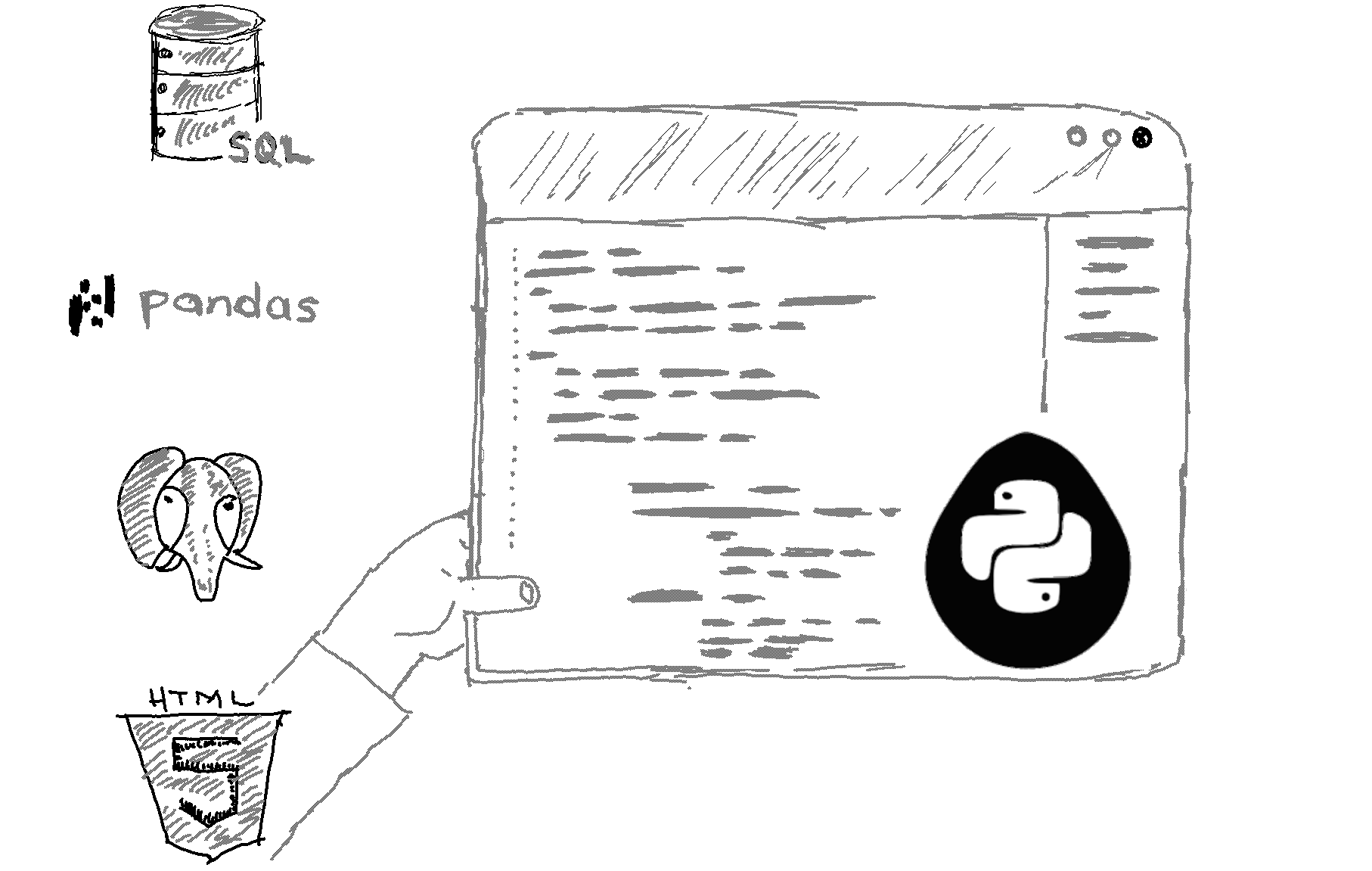# Accessing pandas dataframe columns, rows, and cells

At this point, you know how to load CSV data in Python. In this lesson, you will learn how to access rows, columns, cells, and subsets of rows and columns from a pandas dataframe. Let’s open the CSV file again, but this time we will work smarter. We will not download the CSV from the web manually. We will let Python directly access the CSV download URL.

#### Reading a CSV file from a URL with pandas

`import pandas as pdurl_prefix = "http://"df1 = pd.read_csv(url_prefix + "pythonhow.com/data/income_data.csv")`

The dataframe will be identical to the dataframe we used in the previous lesson. Again, once you have the dataframe loaded on your Jupyter notebook, you can apply operations to your dataframe. Just for reference, here is how the complete dataframe looks like:And before extracting data from the dataframe, it would be a good practice to assign a column with unique values as the index of the dataframe. The State column would be a good choice.

##### Assigning an index column to pandas dataframe:
`df2 = df1.set_index("State", drop = False)`

Note: As you see you needed to store the result in a new dataframe because this is not an in-place operation. Also note that you should set the drop argument to False. If you don’t do that the State column will be deleted so if you set another index later you would lose the State column.

The df2 dataframe would look like this now:Now, let’s extract a subset of the dataframe.

##### Extracting a subset of a pandas dataframe:

Here is the general syntax rule to subset portions of a dataframe:

`df2.loc[startrow:endrow, startcolumn:endcolumn]`

If you can’t wrap your mind around that, here is a neat example that extracts the values for the rows from Alaska through Arkansas for years 2005 to 2007:

`df2.loc["Alaska":"Arkansas","2005":"2007"]`##### Extracting a column of a pandas dataframe:
`df2.loc[: , "2005"]`##### To extract a column, you can also do:
`df2["2005"]`

Note that when you extract a single row or column, you get a one-dimensional object as output. That is called a pandas Series. Whereas, when we extracted portions of a pandas dataframe like we did earlier, we got a two-dimensional DataFrame type of object. Just something to keep in mind for later. So, the formula to extract a column is still the same, but this time we didn’t pass any index name before and after the first colon. Not passing anything tells Python to include all the rows.

##### Extracting a row of a pandas dataframe:
`df2.loc["California", : ]`

##### Extracting specific columns of a pandas dataframe:
`df2[["2005", "2008", "2009"]]`

That would only columns 2005, 2008, and 2009 with all their rows.

##### Extracting specific rows of a pandas dataframe
`df2[1:3]`

That would return the row with index 1, and 2. The row with index 3 is not included in the extract because that’s how the slicing syntax works. Note also that row with index 1 is the second row. Row with index 2 is the third row and so on. If you’re wondering, the first row of the dataframe has an index of 0. That’s just how indexing works in Python and pandas.

##### Extracting a single cell from a pandas dataframe:
`df2.loc["California","2013"]`

##### Note that you can also apply methods to the subsets:
`df2.loc[:,"2005"].mean()`

That, for example, would return the mean income value for year 2005 for all states of the dataframe.

##### Position-based indexing:

Now, sometimes, you don’t have row or column labels. In such case, you will have to rely on position-based indexing, which is implemented with iloc instead of loc:

`df2.iloc[0:3,0:4]`Note that when we used label-based indexing, both the start and the end labels were included in the subset. With position-based slicing, only the start index is included. So, in this case Alabama had an index of 0, Alaska 1, and Arizona 2. The same goes for the columns.

And one more thing you should know about indexing is that when you have labels for either the rows or the columns, and you want to slice a portion of the dataframe. You wouldn’t know whether to use loc or iloc. In this case, you would want to use ix:

`df2.ix[0:3,"2005":"2007"]`

It’s recommended though that you use loc and iloc whenever you can.

Great! Now you know how to access data from your dataframe. You can then use those extracts to perform analysis and visualizations.

Next Lecture